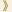HomeShaw Laureates2010Mathematical SciencesPress Release

Mathematical analysis deals with limiting processes such as the approximation of a circle by inscribed regular polygons with increasing numbers of sides (a method used by Archimedes), or the notion of instantaneous velocity used in dynamics. The calculus of Newton and Leibniz provided the machinery for its successful application, from the orbits of planets, to flight of aeroplanes and the devastation of a tsunami.

Underpinning this limiting process is a variety of inequalities, often of a combinatorial nature, whose precise formulation and proof require great insight and ingenuity. The tools and language of analysis form the foundation for vast areas of mathematics, ranging from probability theory and statistical physics to partial differential equations, dynamical systems, combinatorics and number theory.

Jean Bourgain is one of the most brilliant analysts of our times. He has resolved central and long-standing problems in each of the above fields. In doing so he has introduced fundamental techniques many of which have become standard tools in these areas. His work and ideas have greatly enhanced the very fruitful cross fertilizations between all these disciplines.

A prime example of his work is his development of the sum product phenomenon. This is a fundamental combinatorial property which quantifies the relation between the two most basic operations of addition and multiplication. He has used this sum-product theory to resolve problems connected with distribution and counting of symmetries, combinatorics, number theory and solutions of algebraic equations.

More surprisingly, these techniques of Bourgain are intimately related to the very subtle geometry of the Kakeya problem, where a car (idealized as a line segment) is to be reversed in an arbitrarily small area, using an N-point turn with very large N.

In many areas of mathematics and science, random numbers play a key role, but they are in fact hard to produce: tossing a coin is not a practical solution and the coin may be biased. Bourgain has applied his techniques to provide explicit structures that exhibit randomness, and these have important applications in theoretical computer science.

Mathematical Sciences Selection Committee
The Shaw Prize

27 May 2010, Hong Kong